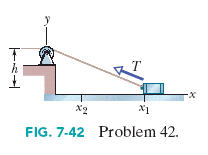# Variable Force

Goatsenator

## Homework Statement

Figure 7-40 shows a cord attached to a cart that can slide along a frictionless horizontal rail aligned along an x axis. The left end of the cord is pulled over a pulley, of negligible mass and friction and at cord height h = 1.4 m, so the cart slides from x1 = 4.0 m to x2 = 2.0 m. During the move, the tension in the cord is a constant 25.0 N. What is the change in the kinetic energy of the cart during the move?## Homework Equations

Delta K = W = Int[ F(x)dx ] from initial position to final position

## The Attempt at a Solution

I let T=25N

So the variable force is in the negative direction but the angle is measured from the negative x direction so it actually = Tcos(180-Θ) = -Tcos(Θ)
And cos(Θ) in terms of x is x/sqrt(x^2+h^2)
T is constant so I pull it out of the integral
Then I integrate from b=2 a=4

-T * Int[{x/sqrt(x^2+h^2)}dx]

=-T [sqrt(x^2+h^2)] from b=2 a=4

I plug it all in and get 300J but it's incorrect. I tried it by myself and couldn't get the answer so I went to my TA and this is how he said to do it, so I don't know what else to do. I'm hoping someone on here can explain this better.

## Answers and Replies

Mentor
I think you need to plug in the values again and recalculate. Your method looks okay, but your final value is not correct.

Goatsenator
Wow... I forgot to take the square root when I calculated.... I apologize for this thread. -_-